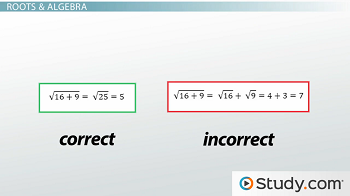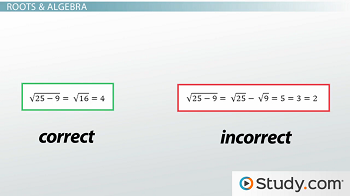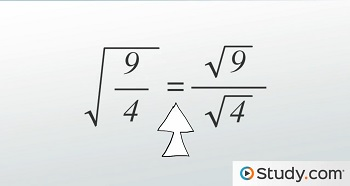# Roots and Powers of Algebraic Expressions

An error occurred trying to load this video.

Try refreshing the page, or contact customer support.

Coming up next: Simplifying Square Roots of Powers in Radical Expressions

### You're on a roll. Keep up the good work!

Replay
Your next lesson will play in 10 seconds
• 0:01 Roots & Exponents
• 0:45 Roots & Algebra
• 2:51 Powers & Algebra
• 4:20 Lesson Summary
Save Save

Want to watch this again later?

Timeline
Autoplay
Autoplay
Speed Speed

#### Recommended Lessons and Courses for You

Lesson Transcript
Instructor: Elizabeth Foster

Elizabeth has been involved with tutoring since high school and has a B.A. in Classics.

In this lesson, you'll learn about some common mistakes that students make when working with roots and powers of algebraic expressions on the SAT and how to make sure you don't fall into the same trap.

## Roots & Exponents

Working with roots and exponents can be tricky at the best of times, but when you start adding in algebraic expressions and factoring, it gets even harder. The bad news is that the test writers on the SAT love to throw in problems that throw students for a loop. But the good news is that by learning how to spot these kinds of problems, you can avoid jumping to the wrong conclusions.

In this lesson, you'll learn how to spot two common traps for students working with roots and powers of algebraic expressions. Both of them revolve around distribution properties and order of operations, or the rule that tells us which operation of a given expression to do first. First, we'll go over how these properties work with roots, and then we'll cover how they're applied to exponents.

## Roots & Algebra

Roots of algebraic equations can be tricky. The key is to remember when you can distribute the radical and when you can't. Here's the rule: only distribute the radical when the operation underneath is multiplication or division - never with addition or subtraction.

When you have addition or subtraction under the radical sign, you have to do the addition or subtraction before you take the root. The square root of x + y is not the same thing as the square root of x + the square root of y.

To illustrate this, let's plug in some real numbers. Let's say x = 16 and y = 9. We'll solve it two ways. On the left is the correct solution and on the right is the incorrect solution.On the left, you first add 16 + 9 to get 25 and then take the square root of that, which is 5. On the right, you can see that if you distribute the radical before doing the addition, you end up with a totally different answer.

We can do the same thing with subtraction: again, on the left, you first subtract 25 - 9 to get 16, and then you take the square root of that to get 4. But if you do it wrong and distribute the radical before subtracting, you end up with 2 as your answer.With addition and subtraction, always do the operation underneath the radical before you start worrying about the radical itself. But with multiplication and division, it's different: the square root of x * y is the same thing as the root of x times the root of y. To illustrate this, we'll plug in some numbers: x = 9 and y = 4.On the left, you take 9 * 4 first to get 36 and then take the square root of that to get 6. On the right, you take the square root of 9 times the square root of 4, which is 3 * 2, which is also 6. As you can see, both of these give you the same answer.

It works the same way with division: the square root of 9/4 is the same thing as the square root of 9 over the square root of 4. So with addition and subtraction, you can't distribute the radical sign. But with multiplication and division, you can.To unlock this lesson you must be a Study.com Member.

### Register to view this lesson

Are you a student or a teacher?

#### See for yourself why 30 million people use Study.com

##### Become a Study.com member and start learning now.
Back
What teachers are saying about Study.com

### Earning College Credit

Did you know… We have over 200 college courses that prepare you to earn credit by exam that is accepted by over 1,500 colleges and universities. You can test out of the first two years of college and save thousands off your degree. Anyone can earn credit-by-exam regardless of age or education level.